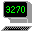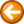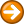Convert - convert a screen position to a row or column or convert a row and column to a screen position\$return = Convert(position,{ROW | COL})

\$return = Convert(row,column,POS)

Where:

 position is the screen position relative to one. row is a  row number. column is a column number.

position, row and/or column may be variables.

The Convert command converts a screen position to a row or column, or it converts a row and column to a screen position. The Convert command  assigns the result of the conversion to the return variable. The upper left-hand corner of the screen is position 1 or  row 1 column 1. The lower right-hand corner of a 24 by 80 screen is position 1920 or row 24 column 80.

Examples

\$row = Convert(81,ROW)

\$col = Convert(81,COL)

\$position = Convert(\$row,\$col,POS)